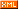# Idiot-maker

:: :: :: :: :: :::: ::291 随笔 :: 0 文章 :: 20 评论 :: 0 引用

https://leetcode.com/problems/repeated-dna-sequences/

All DNA is composed of a series of nucleotides abbreviated as A, C, G, and T, for example: "ACGAATTCCG". When studying DNA, it is sometimes useful to identify repeated sequences within the DNA.

Write a function to find all the 10-letter-long sequences (substrings) that occur more than once in a DNA molecule.

Example:

Input: s = "AAAAACCCCCAAAAACCCCCCAAAAAGGGTTT"

Output: ["AAAAACCCCC", "CCCCCAAAAA"]

class Solution {
public List<String> findRepeatedDnaSequences(String s) {
List<String> res = new ArrayList<String>();

if (s.length() < 11) {
return res;
}

StringBuffer sb = new StringBuffer();
Map<String, Integer> map = new HashMap<String, Integer>();
int index = 0;

while (index <= s.length() - 10) {
String temp = s.substring(index, index + 10);
int count = map.getOrDefault(temp, 0);
if (count == 1) {
res.add(temp);
map.put(temp, count + 1);
} else if (count == 0){
map.put(temp, 1);
}
index++;
}

return res;
}
}

posted on 2018-12-24 23:47 NickyYe 阅读(...) 评论(...) 编辑 收藏